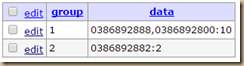## Wednesday, December 21, 2016

### Limiting calls by DIDs for FreePBX users, with dynamic configurable parameters (Repost)So, we had this challenge by our customer to do this as they are using PRI and supporting multiple customers. Each customer needs to be limited to n number of channels on PRI. When they were using analog that was simply straightforward, its a physical line, so nothing much you can do about “limiting” it is limited by design!

The following guide allows you to limit calls based on

• A single DID
• A group of DIDs (in this guide the amount of DIDs per group is limited to 5, add more, improv as you wish)
• Group DIDs will be a union meaning, if you have DID1 and DID2 with limit of 3 calls, at any one time either calls coming to those DIDs are added up and if exceed 3, it will hangup.

Anyway, here’s a quick how-to to give you an idea how to go about it. Improv as you see fit :-)

Requirements: (my system)

1) FreePBX 2.10 or 2.11
2) Asterisk 1.8 or higher
3) Dahdi based PRI or SIP or just about anything with the use of proper declarations (variables)
4) Use MySQL
5) Debian Wheezy
6) Adminer to run a few MySQL tasks such as creating db/tables, editing values in them etc…

So here’s how:

1. Create a database inside MySQL called LIMITER
2. Use adminer and paste the following codes to using the “SQL Command” feature

USE ` LIMITER`;
CREATE TABLE `tbl_didlimiter` (
`group` int(255) NOT NULL AUTO_INCREMENT,
`data` varchar(100) DEFAULT NULL,
PRIMARY KEY (`group`)
) ENGINE=MyISAM DEFAULT CHARSET=latin1;

3. Now, we will create a user superuser with password dbgod00, paste the following codes in SQL Command again

CREATE USER 'superuser'@'localhost' IDENTIFIED BY ‘dbgod00';
GRANT ALL PRIVILEGES ON LIMITER.* TO 'superuser'@'localhost';
FLUSH PRIVILEGES;

4. Now, we edit the dialplan, the most important part! Since i use FreePBX, we use the _custom.conf to add new hacks. So that’s exactly what we are doing here, copy and paste the codes into extensions_custom.conf, like below

#nano /etc/assterisk/extensions_custom.conf

;; DIALPLAN START ;;

;; READ ME FIRST
;; copyleft sanjay@astiostech.com
;; 1. Set trunks to use from-pstn, from-dahdi, from-zaptel OR from-trunk contexts
;; 2. The bold highlights may need to be changed depending on what you see in the
;; channel variables, most cases we see either EXTEN or FROM_DID or even CALLERID(DNID)
;; 3. Be sure this value is available and matching each other, in my case, the value
;; \${EXTEN} eventually matches the value \${CALLERID(DNID)} and they must
;; 4. This only supports one unique DID entry in DB. IF there are multiple entries
;; by mistake or whatever, it will pickout the first result that returns only
;;
;; 5. Feel free to add more G numbers as shown below, right now its just 5
;; 6. Maxdefault is set for global when no DB definitions are found for that DID and its limit,
;; if you do not want blanket settings, simply set MAXDEFAULT to blank (as per default) if you want to set a global
;; limit then set it with MAXDEFAULT which then applies to
all DIDs not set in DB.
;; Only when
there’s a value found in DB then that DB value’s limits overrides maxdefault

[from-pstn-custom]
exten => _X.,1,NoOp(Handling incoming to do cool stuff)
same => n,Set(GROUP()=\${EXTEN})
same => n,Macro(didchoke)

[macro-didchoke]
exten => s,1,NoOp(Checking for incoming limits and applying if needed)
exten => s,n,Set(MAXDEFAULT=””)
exten => s,n,MYSQL(Connect connid localhost superuser dbgod00 LIMITER)
exten => s,n,MYSQL(Query resultid \${connid} SELECT data from tbl_didlimiter where data like '%\${CALLERID(DNID)}%' LIMIT 1)
exten => s,n,MYSQL(Fetch fetchid \${resultid} DBRESULT)
exten => s,n,MYSQL(Clear \${resultid})
exten => s,n,MYSQL(Disconnect \${connid})

exten => s,n,ExecIf(\$["\${DBRESULT}"=""]?Set(DBRESULT=\${CALLERID(DNID)})
exten => s,n,GotoIf(\$["\${DBRESULT}"=""]?exception)

;
exten => s,n,Set(GROUPLIMIT=\${CUT(DBRESULT,:,2)})
exten => s,n,ExecIf(\$["\${GROUPLIMIT}"=""]?Set(GROUPLIMIT=\${MAXDEFAULT})
exten => s,n,GotoIf(\$["\${GROUPLIMIT}"=""]?exception)
;
exten => s,n,Set(DIDS=\${CUT(DBRESULT,:,1)})
exten => s,n,Set(DID1=\${CUT(DIDS,\,,1)})
exten => s,n,Set(DID2=\${CUT(DIDS,\,,2)})
exten => s,n,Set(DID3=\${CUT(DIDS,\,,3)})
exten => s,n,Set(DID4=\${CUT(DIDS,\,,4)})
exten => s,n,Set(DID5=\${CUT(DIDS,\,,5)})
;
exten => s,n,ExecIf(\$["\${DID1}"!=""]?Set(G1=\${GROUP_COUNT(\${DID1})}))
exten => s,n,ExecIf(\$["\${DID2}"!=""]?Set(G2=\${GROUP_COUNT(\${DID2})}))
exten => s,n,ExecIf(\$["\${DID3}"!=""]?Set(G3=\${GROUP_COUNT(\${DID3})}))
exten => s,n,ExecIf(\$["\${DID4}"!=""]?Set(G4=\${GROUP_COUNT(\${DID4})}))
exten => s,n,ExecIf(\$["\${DID5}"!=""]?Set(G5=\${GROUP_COUNT(\${DID5})}))
;
exten => s,n,ExecIf(\$["\${DID1}"=""]?Set(G1=0)
exten => s,n,ExecIf(\$["\${DID2}"=""]?Set(G2=0)
exten => s,n,ExecIf(\$["\${DID3}"=""]?Set(G3=0)
exten => s,n,ExecIf(\$["\${DID4}"=""]?Set(G4=0)
exten => s,n,ExecIf(\$["\${DID5}"=""]?Set(G5=0)
;
exten => s,n,Set(TOTALGGROUPCHANS=\$[\${G1}+\${G2}+\${G3}+\${G4}+\${G5}])
exten => s,n,NoOp(So total channels here are \${TOTALGGROUPCHANS} of GROUPLIMIT of \${GROUPLIMIT})
exten => s,n,GotoIf(\$[\${TOTALGGROUPCHANS} > \${GROUPLIMIT}]?overlimit)
exten => s,n,MacroExit()
;
exten => s,n(overlimit),Busy(20)
exten => s,n,Hangup(16)
exten => s,n,MacroExit()
;
exten => s,n(exception),MacroExit()

;; DIALPLAN END ;;

5. Now, reload asterisk dialplan, be sure to tail the log file to start troubleshooting if things don’t go right.
#asterisk -rx “dialplan reload”
6. Now, edit the DB values and add DIDs like show in example below, use adminer or similar for easy editing
E.g. 1 Format: DID1:3
Where DID1 is DID you wish to limit to 3 channels
E.g. 2 Format: DID1,DID2,DID3,DID4,DID5:3
Where DID1-5 are the DIDs you wish to limit to 3 channels combined

Here’s sample data from my own server!Fire away, test it out…! As usual, appreciate the feedback and ideas to improve! Do let us know how it went for you!